# Switch First & Last Names w/ Commas – Excel & Google Sheets

This tutorial will demonstrate how to switch first and last names in Excel and Google Sheets.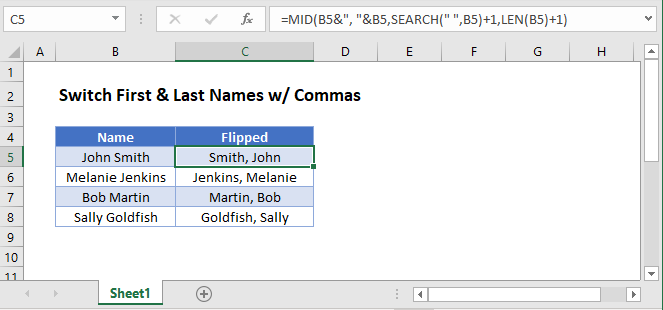## Switch First and Last Names

Typically names are stored in one of two formats:

• Last Name, First Name
• First Name Last Name

Using Excel formulas we can switch the names around.

This formula will switch names from the first format to the second:

``=MID(B3&" "&B3,SEARCH(" ",B3)+1,LEN(B3)-1)``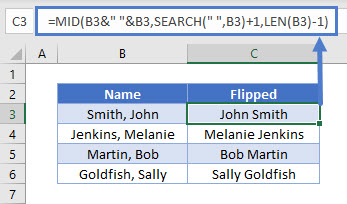We will walkthrough this below.

### SEARCH Function

First, use the SEARCH function to output the position of the space within the name:

``=SEARCH(" ", B3)+1``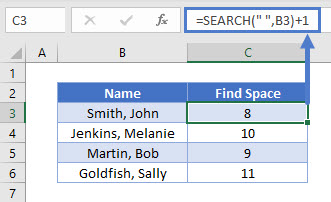Add plus 1 to the formula to get the position of the beginning letter of the first name (skip the space).

### LEN Function

The LEN Function will return the number of characters in a cell.

``=LEN(B3)``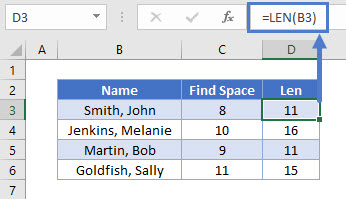### MID Function

Next, use the MID Function to output text from the middle of the name. We will use our previous formulas to determine what text to output.

• The LEN Function helps determine how many characters to return.
``=MID(B3&" "&B3,C3,D3-1)``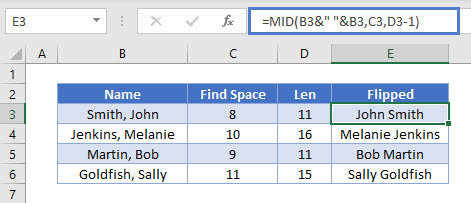Combining these functions yields the original formula.

``=MID(B3&" "&B3,SEARCH(" ",B3)+1,LEN(B3)-1)``

## Switch Last and First Names

You can also flip the text the other way and add in the comma using this formula:

``=MID(B3&", "&B3,SEARCH(" ",B3)+1,LEN(B3)+1)``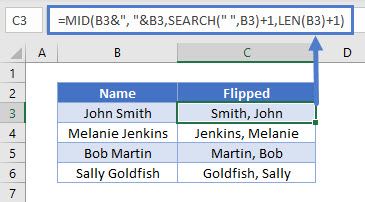## Switch First & Last Names w/ Commas in Google Sheets

These formulas work exactly the same in Google Sheets as in Excel.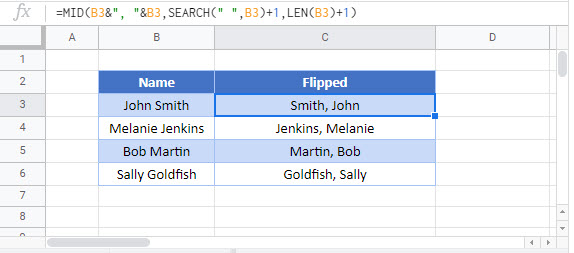### Excel Practice Worksheet

Practice Excel functions and formulas with our 100% free practice worksheets!